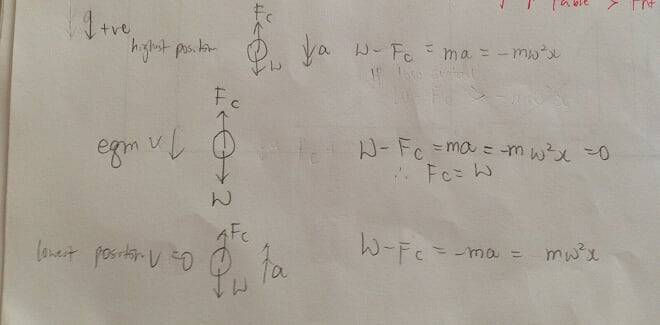# Oscillation (SHM)

## Homework Statement

Some sand is sprinkled onto the cone. The sand oscillates vertically with the frequency of the cone. The amplitude of oscillation of the cone is increased.
At a particular amplitude of oscillation the sand begins to lose contact with the cone.
By considering the forces acting on a grain of sand, explain why this happens.

## Homework Equations

From mark scheme it says "Weight - contact force = m(w^2)x So as x increases, contact force decreases, sand loses contact with cone when contact force = 0"

But I don't understand why it is m(w^2)x, not -m(w^2)x? as I thought they take upward as negative and losing contact only happens at maximum upward displacement position.

## The Attempt at a Solution

Last edited:

From mark scheme it says "Weight - contact force = m(w^2)x So as x increases, contact force decreases, sand loses contact with cone when contact force = 0"

But I don't understand why it is m(w^2)x, not -m(w^2)x? as I thought they take upward as negative and losing contact only happens at maximum upward displacement position.

pl. make out a diagram of oscillations and then mark out the forces acting on sand particle ;perhaps then you can gigure out the contact forces and required centrepetal/centrifugal force .

pl. make out a diagram of oscillations and then mark out the forces acting on sand particle ;perhaps then you can gigure out the contact forces and required centrepetal/centrifugal force .
Yes I did!

Yes I did!
then show it as attachment so that we can get to your problem

then show it as attachment so that we can get to your problemLast edited:
@mystreet123
well the attachment fails to open -perhaps a template will be better so that it can easily open.

@mystreet123
well the attachment fails to open -perhaps a template will be better so that it can easily open.
Can you open it now?

Can you open it now?

yes, thanks

at some amplitude of oscillations of the cone the sand particles leaves the contact with surface

At a particular amplitude of oscillation the sand begins to lose contact with the cone.

From mark scheme it says "Weight - contact force = m(w^2)x S

suppose N is the contact force so N= mg - mw^2.x at any displacement x so when contact force is zero the wt will be balanced by the term on the right hand ;
as i feel mg is down ward so mw^2 .x shoukd be upward.

or think in terms of F(harmonic osc.) = F(Weight) -N
what is wrong in the above picture of forces?

at some amplitude of oscillations of the cone the sand particles leaves the contact with surface

suppose N is the contact force so N= mg - mw^2.x at any displacement x so when contact force is zero the wt will be balanced by the term on the right hand ;
as i feel mg is down ward so mw^2 .x shoukd be upward.
what is wrong in the above picture of forces?
But why I found N-mg = mw^2.x at highest position? if we take upward positive

Because when I use this formula, as x increases, N also increases, instead of becoming zero

mw^2.x at highest position?

in a harmonic oscillation the force which is always a restoring force acts opposite to the displacement

F(osc)= -constantx displacement
if displacement is +ve - force will be negative and just vice-versa
if displacement from mean position is negative then force will be in opposite direction.

•mystreet123
in a harmonic oscillation the force which is always a restoring force acts opposite to the displacement

F(osc)= -constantx displacement
if displacement is +ve - force will be negative and just vice-versa
if displacement from mean position is negative then force will be in opposite direction.